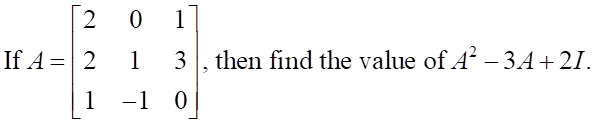IfA-23 | . then find the value of.f-3A + 2111 -1 0

Questionhelp_outlineImage TranscriptioncloseIfA-2 3 | . then find the value of.f-3A + 21 1 1 -1 0 fullscreen
Step 1

We first find A2, for which we multiply A with itself. We perform matrix multiplication in which we multiply rows of first matrix with columns of second matrix element by element and add.

Step 2

Let us now find 3A, for which multiply each element of A by 3.

Step 3

Now, to find 2I we consider the identity matrix of order 3x3 and multiply its each element by 2. We take order 3x3 to match with the order of A2...

Want to see the full answer?

See Solution

Want to see this answer and more?

Our solutions are written by experts, many with advanced degrees, and available 24/7

See Solution
Tagged in

Algebra